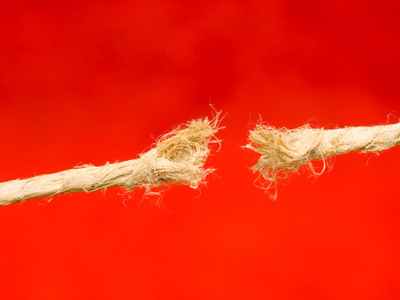If a piece of rope measures 50cm and I cut off a piece measuring 20cm, how much rope is left?

# Subtraction Problems

This Math quiz is called 'Subtraction Problems' and it has been written by teachers to help you if you are studying the subject at elementary school. Playing educational quizzes is a fun way to learn if you are in the 1st or 2nd grade - aged 6 to 8.

It costs only \$12.50 per month to play this quiz and over 3,500 others that help you with your school work. You can subscribe on the page at Join Us

Solving subtraction problems means being able to recognize key vocabulary such as 'take away' or 'minus' and calculate accordingly. This quiz will help children to become familiar with these words and help them to solve subtraction problems.

Can you solve the subtraction problems?

1.
Kim is given £20 for her birthday. Jake gets £12 less than this. How much does Jake get?
£20
£12
£10
£8
Counting back from the larger number to the smaller one is useful when finding 'less than'
2.
I have 12 books and lend 4 to my friend. How many books do I have left?
4
8
6
10
12 - 4 = 8 so 8 books are left
3.
18 minus 12 = 10 - ?
4
6
3
5
The two subtractions should give the same answer, which is 6
4.
What is 9 subtracted from 18?
11
10
8
9
Subtract means to take away
5.
What is 15 minus 9?
6
8
9
5
Minus means to take away
6.
I buy 24 cakes. My brother eats 6, I eat 4 and mom eats 7. How many cakes are left?
9
6
8
7
17 cakes are eaten, so 24 - 17 = 7
7.
A piece of rope measures 90cm. I cut off a piece measuring 25cm and another piece measuring 20cm. How much rope is left?
45cm
40cm
50cm
55cm
90 - 25 = 65.
65 - 20 = 45
8.
Sarah walks 15 miles. Fran walks 9 miles. How much further than Fran has Sarah walked?
8 miles
7 miles
5 miles
6 miles
15 - 9 = 6
9.
What is the difference between 13 and 22?
One is odd, one is even
One is larger than the other
The difference is 9
One is smaller then the other
The other 3 options are true but in math 'difference' means to take away. To find the difference between two numbers, subtract the smaller from the larger
10.
I buy a tray of 20 eggs. Omelets need 2 eggs each. How many eggs will be left if I make 5 omelets?
11
9
10
20
20 - (5 x 2) = 10
Author:  Angela Smith